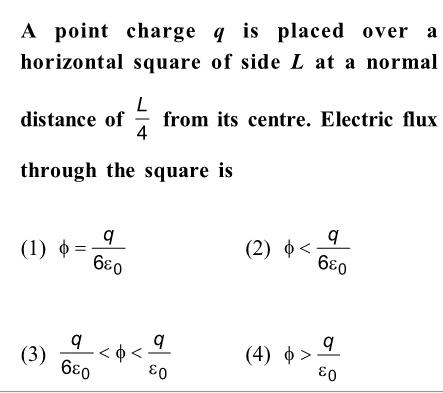Concept Videos :-

#22 | Electric Flux
#23 | Gauss Theorem
#24 | Charged Infinite Wire (Gauss Theorem)
#25 | Charged Infinite Sheet (Gauss Theorem)
#26 | Charged Thin Conducting Shell (Gauss Theorem)
#27 | Solid Non Conducting Sphere : Gauss Theorem
#28 | Solid Sphere With Cavity : Gauss Theorem
#29 | Early Model of Atom (Gauss Theorem)
#30 | EFI Inside a Metal (Gauss Theorem)
#31 | Faraday Cages (Gauss Theorem)

Concept Questions :-

Gauss law

(3)

To use Gauss Law, we have to consider a Gaussian surface whose the given square is a part of.

Using Gauss Law, we can find flux through the Gaussian surface and then we can divide the flux through each square.

Imagine a case, when a point charge q is placed at the center of a cube of side L.

 $⇒$ Flux passing through cube=$\mathrm{q}/{\in }_{0}$ $⇒$ By symmetry, flux passing through each square =$\mathrm{q}/6{\in }_{0}$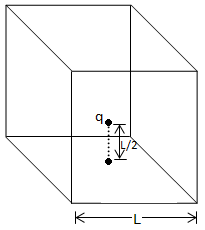When the charge is placed closer to the square face i.e. at L/2, flux passing through square face will be more.
So:- $\frac{\mathrm{q}}{6{\in }_{0}}<\varnothing <\frac{\mathrm{q}}{{\in }_{0}}$

Difficulty Level:

• 39%
• 13%
• 37%
• 12%

A solid conducting sphere of radius a has a net positive charge 2Q. A conducting spherical shell of inner radius b and outer radius c is concentric with the solid sphere and has a net charge –Q. The surface charge density on the inner and outer surfaces of the spherical shell will be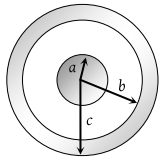(1) $-\frac{2Q}{4\pi {b}^{2}},\frac{Q}{4\pi {c}^{2}}$

(2) $-\frac{Q}{4\pi {b}^{2}},\frac{Q}{4\pi {c}^{2}}$

(3) $0,\frac{Q}{4\pi {c}^{2}}$

(4) None of the above

Concept Videos :-

#22 | Electric Flux
#23 | Gauss Theorem
#24 | Charged Infinite Wire (Gauss Theorem)
#25 | Charged Infinite Sheet (Gauss Theorem)
#26 | Charged Thin Conducting Shell (Gauss Theorem)
#27 | Solid Non Conducting Sphere : Gauss Theorem
#28 | Solid Sphere With Cavity : Gauss Theorem
#29 | Early Model of Atom (Gauss Theorem)
#30 | EFI Inside a Metal (Gauss Theorem)
#31 | Faraday Cages (Gauss Theorem)

Concept Questions :-

Gauss law

(1) Surface charge density (σ)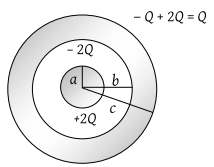So ${\sigma }_{inner}=\frac{-2Q}{\text{\hspace{0.17em}}4\pi {b}^{2}}$ and ${\sigma }_{Outer}=\frac{Q}{\text{\hspace{0.17em}}4\pi {c}^{2}}$

Difficulty Level:

• 48%
• 20%
• 25%
• 9%

A cylinder of radius R and length L is placed in a uniform electric field E parallel to the cylinder axis. The total flux for the surface of the cylinder is given by

(1) $2\pi {R}^{2}E$

(2) $\pi {R}^{2}/E$

(3) $\left(\pi {R}^{2}-\pi R\right)/E$

(4) Zero

Concept Videos :-

#22 | Electric Flux
#23 | Gauss Theorem
#24 | Charged Infinite Wire (Gauss Theorem)
#25 | Charged Infinite Sheet (Gauss Theorem)
#26 | Charged Thin Conducting Shell (Gauss Theorem)
#27 | Solid Non Conducting Sphere : Gauss Theorem
#28 | Solid Sphere With Cavity : Gauss Theorem
#29 | Early Model of Atom (Gauss Theorem)
#30 | EFI Inside a Metal (Gauss Theorem)
#31 | Faraday Cages (Gauss Theorem)

Concept Questions :-

Gauss law

(4) Flux through surface A ${\phi }_{A}=E×\pi {R}^{2}$ and ${\phi }_{B}=-E×\pi {R}^{2}$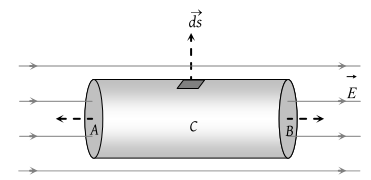Flux through curved surface C $=\int \stackrel{\to }{E}.\stackrel{\to }{ds}=\int E\text{\hspace{0.17em}}ds\mathrm{cos}{90}^{o}$ = 0

∴ Total flux through cylinder $={\phi }_{A}+{\phi }_{B}+{\phi }_{C}$ = 0

Difficulty Level:

• 25%
• 7%
• 9%
• 61%

An electric charge is placed at the centre of a cube of side α. The electric flux on one of its faces will be

(1) $\frac{q}{6{\epsilon }_{0}}$

(2) $\frac{q}{{\epsilon }_{0}{a}^{2}}$

(3) $\frac{q}{4\pi {\epsilon }_{0}{a}^{2}}$

(4) $\frac{q}{{\epsilon }_{0}}$

Concept Videos :-

#22 | Electric Flux
#23 | Gauss Theorem
#24 | Charged Infinite Wire (Gauss Theorem)
#25 | Charged Infinite Sheet (Gauss Theorem)
#26 | Charged Thin Conducting Shell (Gauss Theorem)
#27 | Solid Non Conducting Sphere : Gauss Theorem
#28 | Solid Sphere With Cavity : Gauss Theorem
#29 | Early Model of Atom (Gauss Theorem)
#30 | EFI Inside a Metal (Gauss Theorem)
#31 | Faraday Cages (Gauss Theorem)

Concept Questions :-

Gauss law

(1) By Gauss's theorem.

Imagine a case when a point charge q is placed at the centre of a cube of side L.

 $⇒$ Flux passing through cube=$\mathrm{q}/{\in }_{0}$ $⇒$ By symmetry, flux passing through each square =$\mathrm{q}/6{\in }_{0}$Difficulty Level:

• 81%
• 5%
• 7%
• 9%

Total electric flux coming out of a unit positive charge put in air is

(1) ${\epsilon }_{0}$

(2) ${\epsilon }_{0}^{-1}$

(3) ${\left(4p{\epsilon }_{0}\right)}^{-1}$

(4) $4\pi {\epsilon }_{0}$

Concept Videos :-

#22 | Electric Flux
#23 | Gauss Theorem
#24 | Charged Infinite Wire (Gauss Theorem)
#25 | Charged Infinite Sheet (Gauss Theorem)
#26 | Charged Thin Conducting Shell (Gauss Theorem)
#27 | Solid Non Conducting Sphere : Gauss Theorem
#28 | Solid Sphere With Cavity : Gauss Theorem
#29 | Early Model of Atom (Gauss Theorem)
#30 | EFI Inside a Metal (Gauss Theorem)
#31 | Faraday Cages (Gauss Theorem)

Concept Questions :-

Gauss law

(2)Total flux coming out from unit charge $=\stackrel{\to }{E}.d\stackrel{\to }{s}=\frac{1}{{\epsilon }_{0}}×1={\epsilon }_{0}^{-1}$

Difficulty Level:

• 14%
• 73%
• 7%
• 7%

A cube of side l is placed in a uniform field E, where $E=E\stackrel{^}{i}$. The net electric flux through the cube is

(1) Zero

(2) l2 E

(3) 4 l2 E

(4) 6 l2 E

Concept Videos :-

#22 | Electric Flux
#23 | Gauss Theorem
#24 | Charged Infinite Wire (Gauss Theorem)
#25 | Charged Infinite Sheet (Gauss Theorem)
#26 | Charged Thin Conducting Shell (Gauss Theorem)
#27 | Solid Non Conducting Sphere : Gauss Theorem
#28 | Solid Sphere With Cavity : Gauss Theorem
#29 | Early Model of Atom (Gauss Theorem)
#30 | EFI Inside a Metal (Gauss Theorem)
#31 | Faraday Cages (Gauss Theorem)

Concept Questions :-

Gauss law

(1) As there is no charge residing inside the cube, hence net flux is zero.

Difficulty Level:

• 68%
• 11%
• 7%
• 15%

A charge q is placed at the centre of the open end of cylindrical vessel. The flux of the electric field through the surface of the vessel is

(1) Zero

(2) $\frac{q}{{\epsilon }_{0}}$

(3) $\frac{q}{2{\epsilon }_{0}}$

(4) $\frac{2q}{{\epsilon }_{0}}$

Concept Videos :-

#22 | Electric Flux
#23 | Gauss Theorem
#24 | Charged Infinite Wire (Gauss Theorem)
#25 | Charged Infinite Sheet (Gauss Theorem)
#26 | Charged Thin Conducting Shell (Gauss Theorem)
#27 | Solid Non Conducting Sphere : Gauss Theorem
#28 | Solid Sphere With Cavity : Gauss Theorem
#29 | Early Model of Atom (Gauss Theorem)
#30 | EFI Inside a Metal (Gauss Theorem)
#31 | Faraday Cages (Gauss Theorem)

Concept Questions :-

Gauss law

(3) To apply Gauss's theorem it is essential that charge should be placed inside a closed surface. So imagine another similar cylindrical vessel above it as shown in figure (dotted).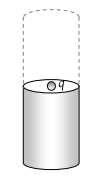Difficulty Level:

• 40%
• 19%
• 39%
• 3%

According to Gauss’ Theorem, electric field of an infinitely long straight wire is proportional to

(1) r

(2) $\frac{1}{{r}^{2}}$

(3) $\frac{1}{{r}^{3}}$

(4) $\frac{1}{r}$

Concept Videos :-

#22 | Electric Flux
#23 | Gauss Theorem
#24 | Charged Infinite Wire (Gauss Theorem)
#25 | Charged Infinite Sheet (Gauss Theorem)
#26 | Charged Thin Conducting Shell (Gauss Theorem)
#27 | Solid Non Conducting Sphere : Gauss Theorem
#28 | Solid Sphere With Cavity : Gauss Theorem
#29 | Early Model of Atom (Gauss Theorem)
#30 | EFI Inside a Metal (Gauss Theorem)
#31 | Faraday Cages (Gauss Theorem)

Concept Questions :-

Gauss law

(4) $e=\frac{\lambda }{2\pi {\epsilon }_{0}r}$$E\propto \frac{1}{r}$

Difficulty Level:

• 6%
• 20%
• 6%
• 70%

Electric charge is uniformly distributed along a long straight wire of radius 1mm. The charge per cm length of the wire is Q coulomb. Another cylindrical surface of radius 50 cm and length 1m symmetrically encloses the wire as shown in the figure. The total electric flux passing through the cylindrical surface is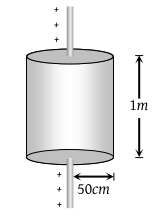(1) $\frac{Q}{{\epsilon }_{0}}$

(2) $\frac{100Q}{{\epsilon }_{0}}$

(3) $\frac{10Q}{\left(\pi {\epsilon }_{0}\right)}$

(4) $\frac{100Q}{\left(\pi {\epsilon }_{0}\right)}$

Concept Videos :-

#22 | Electric Flux
#23 | Gauss Theorem
#24 | Charged Infinite Wire (Gauss Theorem)
#25 | Charged Infinite Sheet (Gauss Theorem)
#26 | Charged Thin Conducting Shell (Gauss Theorem)
#27 | Solid Non Conducting Sphere : Gauss Theorem
#28 | Solid Sphere With Cavity : Gauss Theorem
#29 | Early Model of Atom (Gauss Theorem)
#30 | EFI Inside a Metal (Gauss Theorem)
#31 | Faraday Cages (Gauss Theorem)

Concept Questions :-

Gauss law

(2) Charge enclosed by cylindrical surface (length 100 cm) is Qenc = 100Q.

By applying Gauss's law $\phi =\frac{1}{{\epsilon }_{0}}\left({Q}_{enc.}\right)=\frac{1}{{\epsilon }_{0}}\left(100Q\right)$

Difficulty Level:

• 25%
• 58%
• 9%
• 10%

The S.I. unit of electric flux is

(1) Weber

(2) Newton per coulomb

(3) Volt × metre

(4) Joule per coulomb

Concept Videos :-

#22 | Electric Flux
#23 | Gauss Theorem
#24 | Charged Infinite Wire (Gauss Theorem)
#25 | Charged Infinite Sheet (Gauss Theorem)
#26 | Charged Thin Conducting Shell (Gauss Theorem)
#27 | Solid Non Conducting Sphere : Gauss Theorem
#28 | Solid Sphere With Cavity : Gauss Theorem
#29 | Early Model of Atom (Gauss Theorem)
#30 | EFI Inside a Metal (Gauss Theorem)
#31 | Faraday Cages (Gauss Theorem)

Concept Questions :-

Gauss law

(3) S.I. unit of electric flux is $\frac{N×{m}^{2}}{C}=\frac{J×m}{C}$ = volt × m.

Difficulty Level:

• 28%
• 14%
• 51%
• 8%